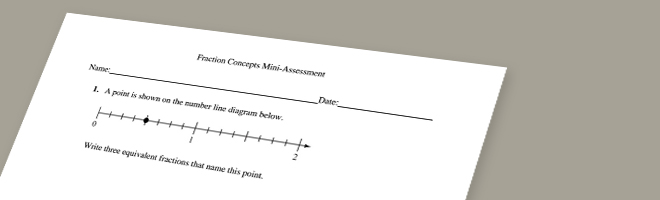• 02/25/15   |   Adjusted: 10/09/19   |   2 files# Fraction Concepts Mini-Assessment

Author: Student Achievement Partners

• Description
• Files

This mini-assessment is designed to illustrate some of the fraction concepts listed in clusters 4.NF.A and 4.NF.B, which set an expectation for students to deepen their understanding of fraction equivalence and ordering, and to develop their understanding of operations on fractions. This mini-assessment is designed for teachers to use either in the classroom, for self-learning, or in professional development settings to:

• Evaluate students’ understanding of aspects of 4.NF.A and 4.NF.B in order to prepare to teach this material or to check for student ability to demonstrate understanding and apply these concepts;
• Gain knowledge about assessing conceptual understanding of fraction equivalence, ordering, and operations; and
• Illustrate CCSS-aligned assessment problems.

• Making the Shifts

How does this mini-assessment exemplify the instructional Shifts required by CCSSM?

 Focus Belongs to the major work of fourth grade Coherence Extends grade 3 understandings of fractions and operations on whole numbers Rigor Conceptual Understanding: primary in this mini-assessment Procedural Skill and Fluency: not targeted in this mini-assessment Application: secondary in this mini-assessment
• Noteworthy features of this resource
• Mathematically:
• Uses context to help students attach meaning to the operation of multiplication when multiplying a fraction by a whole number
• Includes fractions greater than 1 to highlight the expectations of the Standards
• Reinforces that a fraction is a number by showing fractions on number line diagrams
• Extends grade 3 fraction understandings to new denominators of 5, 10, 12, and 100
• As a mini-assessment:
• Assesses important concepts directly, such as knowing any fraction $\frac{a}{b}$ is equivalent to any fraction $\frac{n×a}{n×b}$
• Mixes short conceptual problems with problems that require longer chains of reasoning
• Offers sample solution methods and item-level analyses in the answer key to support teachers using student data from the assessment